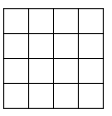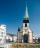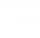# Triangle in a square

In a square ABCD with side a = 6 cm, point E is the center of side AB and point F is the center of side BC. Calculate the size of all angles of the triangle DEF and the lengths of its sides.

Correct result:

d =  2.121 cm
e =  6.708 cm
f =  6.708 cm
E =  71.562 °
F =  71.562 °
D =  36.876 °

#### Solution:

$a=6 \ \text{cm} \ \\ d^2=(a/2)^2 + (a/2)^2 \ \\ \ \\ d=a/\sqrt{ 8 }=6/\sqrt{ 8 }=2.121 \ \text{cm}$
$e^2=a^2 + (a/2)^2=6^2 + (6/2)^2=45 \ \\ \ \\ e=\sqrt{ 1+1/4 } \cdot \ a=\sqrt{ 1+1/4 } \cdot \ 6=3 \ \sqrt{ 5 }=6.708 \ \text{cm}$
$f=e=6.7082=\dfrac{ 1677 }{ 250 }=6.708 \ \text{cm}$
$\angle FEB=45 \ ^\circ \ \\ \ \\ \sin AED=a/e \ \\ \ \\ AED=\dfrac{ 180^\circ }{ \pi } \cdot \arcsin(a/e)=\dfrac{ 180^\circ }{ \pi } \cdot \arcsin(6/6.7082) \doteq 63.4384 \ \\ \ \\ E=180 - AED - \angle FEB=180 - 63.4384 - 45=71.562 ^\circ =71^\circ 33'42"$
$F=E=71.5616=71.562 ^\circ =71^\circ 33'43"$
$D=180-F-E=180-71.562-71.5616=\dfrac{ 9219 }{ 250 }=36.876 ^\circ =36^\circ 52'34"$Our examples were largely sent or created by pupils and students themselves. Therefore, we would be pleased if you could send us any errors you found, spelling mistakes, or rephasing the example. Thank you!

Leave us a comment of this math problem and its solution (i.e. if it is still somewhat unclear...):Be the first to comment!Tips to related online calculators
Pythagorean theorem is the base for the right triangle calculator.

#### You need to know the following knowledge to solve this word math problem:

We encourage you to watch this tutorial video on this math problem:

## Next similar math problems:

• Coordinates of square verticesI have coordinates of square vertices A / -3; 1/and B/1; 4 /. Find coordinates of vertices C and D, C 'and D'. Thanks Peter.
• Trapezoid MOThe rectangular trapezoid ABCD with right angle at point B, |AC| = 12, |CD| = 8, diagonals are perpendicular to each other. Calculate the perimeter and area of ​​the trapezoid.
• Regular 5-gonCalculate area of the regular pentagon with side 7 cm.
• Tetrahedral pyramidDetermine the surface of a regular tetrahedral pyramid when its volume is V = 120 and the angle of the sidewall with the base plane is α = 42° 30´.
• IS triangleCalculate interior angles of the isosceles triangle with base 40 cm and legs 22 cm long.
• Traffic signThere is a traffic sign for climbing on the road with an angle of 7%. Calculate at what angle the road rises (falls).
• Two forcesTwo forces with magnitudes of 25 and 30 pounds act on an object at angles of 10° and 100° respectively. Find the direction and magnitude of the resultant force. Round to two decimal places in all intermediate steps and in your final answer.
• What percentageWhat percentage of the Earth’s surface is seen by an astronaut from a height of h = 350 km. Take the Earth as a sphere with the radius R = 6370 km
• Right triangleCalculate the missing side b and interior angles, perimeter and area of ​​a right triangle if a=10 cm and hypotenuse c = 16 cm.
• TreeBetween points A and B is 50m. From A we see a tree at an angle 18°. From point B we see the tree in three times bigger angle. How tall is a tree?
• Power line poleFrom point A, the power line pole is seen at an angle of 18 degrees. From point B to which we get when going from point A 30m away from the column at an angle of 10 degrees. Find the height of the power pole.
• Ratio iso triangleThe ratio of the sides of an isosceles triangle is 7:6:7 Find the base angle to the nearest answer correct to 3 significant figure.
• The aspect ratioThe aspect ratio of the rectangular triangle is 13: 12: 5. Calculate the internal angles of the triangle.
• Church towerArchdeacon church in Usti nad Labem has diverted tower by 186 cm. The tower is 65 m high. Calculate the angle by which the tower is tilted. Result write in degree's minutes.
• The rescue helicopterThe rescue helicopter is above the landing site at a height of 180m. The site of the rescue operation can be seen from here at a depth angle of 52° 40 '. How far will the helicopter land from the rescue site?
• Sphere in coneA sphere of radius 3 cm desribe cone with minimum volume. Determine cone dimensions.
• Q-ExamIf tg α = 0.9, Calculating sin α, cos α, cotg α .